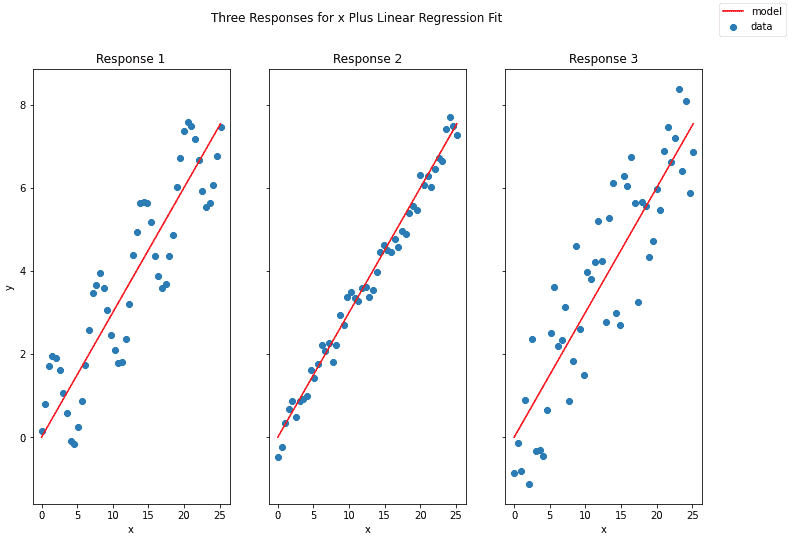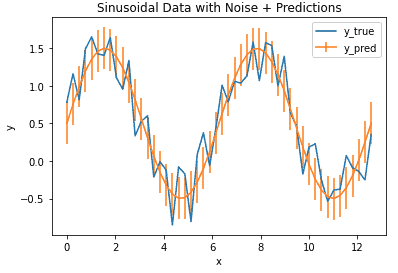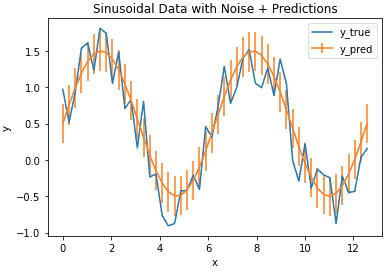# Regression

This category groups articles on the subject of regression. Each post focuses on either a specific regression algorithm, or a tool used when tackling a regression problem. The emphasis here is on understanding these models and techniques at a technical level. Here you will learn to build regression models in Python from scratch.## Coefficient of Determination

Coefficient of Determination Introduction The Coefficient of Determination is a metric for evaluating the goodness of fit for a linear regression model. This quantity is often defined as:    (1) where is the sum of squared errors, and is the sum of squared total variance. Let’s define these, along with the sum of squared regression …## Mean Squared Error

Mean Squared Error Introduction In this post we’ll cover the Mean Squared Error (MSE), arguably one of the most popular error metrics for regression analysis. The MSE is expressed as:    (1) where are the model output and are the true values. The summation is performed over individual data points available in our sample. The advantage …## Mean Absolute Error

Mean Absolute Error Introduction With any machine learning project, it is essential to measure the performance of the model. What we need is a metric to quantify the prediction error in a way that is easily understandable to an audience without a strong technical background. For regression problems, the Mean Absolute Error (MAE) is just such …## Build a Decision Tree in Python from Scratch

Build a Decision Tree in Python from Scratch In this post, we will build a CART Decision Tree model in Python from scratch. We will start with the foundational principals, and work straight through to implementation in code. Both classification and regression examples will be included. Motivation to Build a Decision Tree Model Decision Trees are …## Implement Linear Regression in Python from Scratch

Implement Linear Regression in Python from Scratch For this post, I will implement Linear Regression in Python from scratch. We will start with the fundamental assumptions and mathematical foundations, and work straight through to implementation in Python.   Motivation for Linear Regression It is no secret that the past few years have seen a considerable rise in …